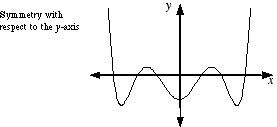index: click on a letter A B C D E F G H I J K L M N O P Q R S T U V W X Y Z A to Z index index: subject areas numbers & symbols sets, logic, proofs geometry algebra trigonometry advanced algebra & pre-calculus calculus advanced topics probability & statistics real world applications multimedia entrieswww.mathwords.com about mathwords website feedback

 Symmetric about the y-axis Symmetric across the y-axis Symmetric with Respect to the y-axis Describes a graph that is left unchanged when reflected across the y-axis.See also